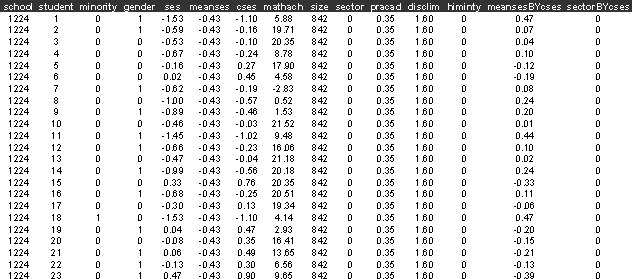Example C1 Continuous response model

The data we use in this example are a sub-sample from the 1982 High School and Beyond Survey (Raudenbush, Bryk, 2002), and include information on 7,185 students nested within 160 schools: 90 public and 70 Catholic.  Sample sizes vary from 14 to 67 students per school.

Reference

Raudenbush, S.W., Bryk, A.S., 2002, Heirarchical Linear Models, Thousand Oaks, CA. Sage

Data description

Number of observations (rows): 7185

Number of variables (columns): 15

The variables include the following:

school=school identifier

student=student identifier

minority =1 if student is from an ethnic minority, 0 = other)

gender = 1 if student is female, 0 otherwise

ses = a standardized scale constructed from variables measuring parental education, occupation, and income, socio economic status

meanses = mean of the SES values for the students in this school

mathach= a measure of the students mathematics achievement

size = school enrolment

sector =1 if  school is from the Catholic sector, 0 = public

pracad = proportion of students in the academic track

disclim = a scale measuring disciplinary climate

himnty =1 if more than 40% minority enrolment, 0 if less than 40%)

The first few lines of hsb.dat look likeSabre commands

out hsb1.log

trace hsb1.trace

data school student minority gender ses meanses cses mathach size sector &

pracad desclim himinty meansesbycses sectorbycses

read hsb.dat

case school

yvar mathach

family g

constant cons

lfit cons

dis m

dis e

mass 64

fit cons

dis m

dis e

lfit meanses cons

dis m

dis e

fit meanses cons

dis m

dis e

stop

Sabre log file

<S> trace hsb1.trace

<S> data school student minority gender ses meanses cses mathach size sector &

<S>      pracad desclim himinty meansesbycses sectorbycses

<S> read hsb.dat

7185 observations in dataset

<S> case school

<S> yvar mathach

<S> family g

<S> constant cons

<S> lfit cons

Iteration       Log. lik.       Difference

__________________________________________

1          -24049.866

<S> dis m

X-vars            Y-var

______________________________

cons              mathach

Univariate model

Standard linear

Number of observations             =    7185

X-var df           =     1

Log likelihood =     -24049.866     on    7184 residual degrees of freedom

<S> dis e

Parameter              Estimate         Std. Err.

___________________________________________________

cons                    12.748          0.81145E-01

sigma                   6.8782

<S> mass 64

<S> fit cons

Initial Homogeneous Fit:

Iteration       Log. lik.       Difference

__________________________________________

1          -24049.866

Iteration       Log. lik.         Step      End-points     Orthogonality

length    0          1      criterion

________________________________________________________________________

1          -23771.600        1.0000    fixed  fixed       448.87

2          -23695.571        1.0000    fixed  fixed       835.31

3          -23652.804        1.0000    fixed  fixed       688.55

4          -23616.853        1.0000    fixed  fixed       404.57

5          -23590.245        1.0000    fixed  fixed       243.42

6          -23572.704        1.0000    fixed  fixed       101.08

7          -23559.124        1.0000    fixed  fixed       43.816

8          -23557.935        1.0000    fixed  fixed       31.439

9          -23557.905        1.0000    fixed  fixed       29.654

10          -23557.905        1.0000    fixed  fixed

<S> dis m

X-vars            Y-var             Case-var

________________________________________________

cons              mathach           school

Univariate model

Standard linear

Gaussian random effects

Number of observations             =    7185

Number of cases                    =     160

X-var df           =     1

Sigma df           =     1

Scale df           =     1

Log likelihood =     -23557.905     on    7182 residual degrees of freedom

<S> dis e

Parameter              Estimate         Std. Err.

___________________________________________________

cons                    12.637          0.24359

sigma                   6.2569          0.52794E-01

scale                   2.9246          0.18257

<S> lfit meanses cons

Iteration       Log. lik.       Difference

__________________________________________

1          -23598.190

<S> dis m

X-vars            Y-var

______________________________

cons              mathach

meanses

Univariate model

Standard linear

Number of observations             =    7185

X-var df           =     2

Log likelihood =     -23598.190     on    7183 residual degrees of freedom

<S> dis e

Parameter              Estimate         Std. Err.

___________________________________________________

cons                    12.713          0.76215E-01

meanses                 5.7168          0.18429

sigma                   6.4596

<S> fit meanses cons

Initial Homogeneous Fit:

Iteration       Log. lik.       Difference

__________________________________________

1          -23598.190

Iteration       Log. lik.         Step      End-points     Orthogonality

length    0          1      criterion

________________________________________________________________________

1          -23502.667        1.0000    fixed  fixed       260.90

2          -23489.442        1.0000    fixed  fixed       200.22

3          -23483.785        1.0000    fixed  fixed       181.86

4          -23481.131        1.0000    fixed  fixed       180.44

5          -23480.072        1.0000    fixed  fixed       180.76

6          -23479.711        1.0000    fixed  fixed       88.600

7          -23479.554        1.0000    fixed  fixed       54.074

8          -23479.554        1.0000    fixed  fixed       53.557

9          -23479.554        1.0000    fixed  fixed

<S> dis m

X-vars            Y-var             Case-var

________________________________________________

cons              mathach           school

meanses

Univariate model

Standard linear

Gaussian random effects

Number of observations             =    7185

Number of cases                    =     160

X-var df           =     2

Sigma df           =     1

Scale df           =     1

Log likelihood =     -23479.554     on    7181 residual degrees of freedom

<S> dis e

Parameter              Estimate         Std. Err.

___________________________________________________

cons                    12.650          0.14834

meanses                 5.8629          0.35917

sigma                   6.2576          0.52800E-01

scale                   1.6103          0.12314

<S> stop